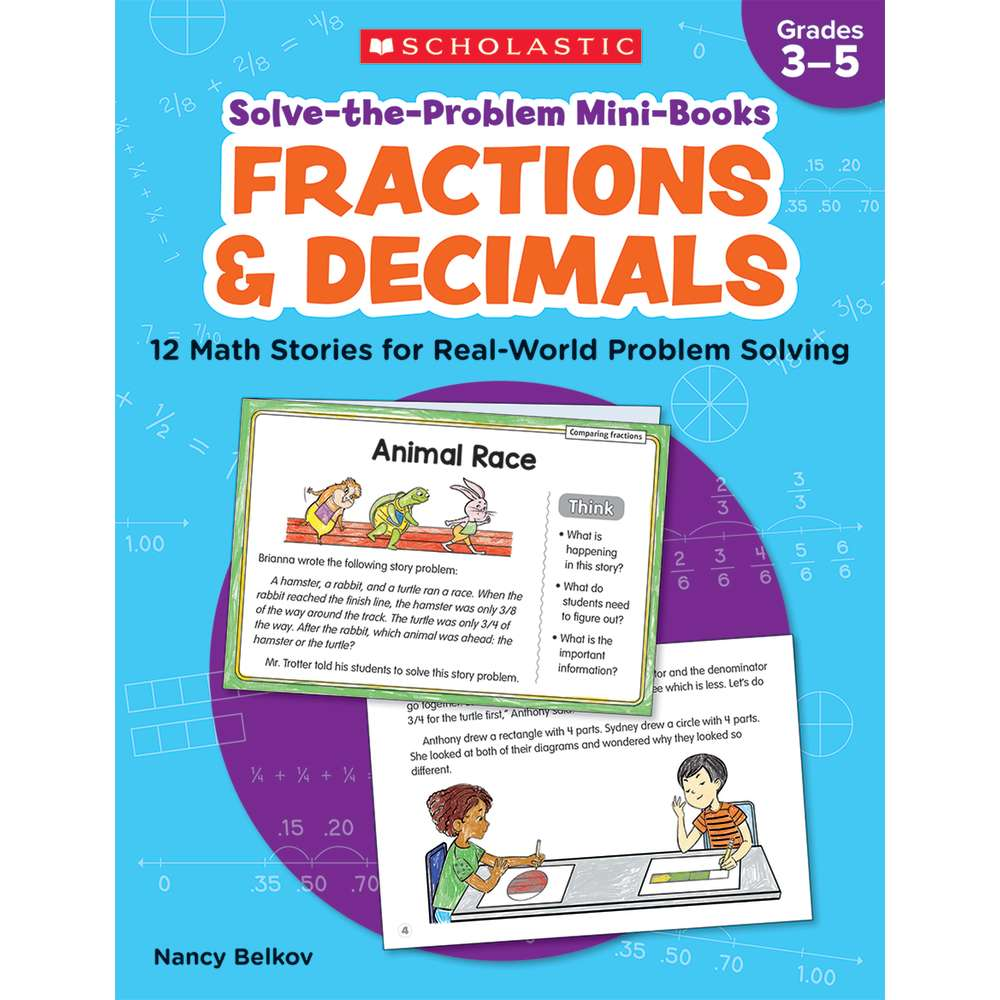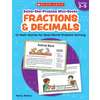•# Solve-the-Problem Mini Books: Fractions & Decimals

Product Number: SC-736590
##### Common Core
\$14.99
Research shows that an effective way to teach math concepts is through problem solving. The mini-stories in this book model h...
Quantity

Research shows that an effective way to teach math concepts is through problem solving. The mini-stories in this book model how students can solve math problems by working together to ask questions, share ideas and strategies, apply prior knowledge to find solutions, and more. Each story comes with follow-up practice problems. Skills include comparing fractions, finding equivalent fractions, using decimal equivalents to compare fractions, adding and subtracting fractions, and more.
SC-736590

### Data sheet

Manufacturer
Scholastic Teaching Solutions